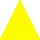# SDL2_gfx: texturedPolygon - how to rotate a textured triangle?

Hello,

I am currently trying to render some polygons with a texture. I’ve already succesfully rendered a circle with a football texture but struggle to render a triangle with a texture.
It seems that I am not calculating (or setting?) the parameter values texture_dx and texture_dy properly.

What I get is this (I am using Box2D for collision/physics):

As you can see, the texture is not exactly on the rendered object, it sometimes “overlaps” and takes the other parts to. The Image is a simple yellow triangle with a white background:What i want is that it only draws the yellow stuff, and not the white (I know I could just make the whole image yellow but I want to use this method to render triangle-sprites later e.g. spikes).

code:

``````/*1. get vertices and transfer them to pixels*/

b2Vec2 vertices_triangle;
for(int i = 0; i < 3; i++)
{
vertices_triangle[i] = triangle_body->GetWorldPoint(triangleShape.GetVertex(i));
}

// Calculate "render"vertices of the triangle
b2Vec2 A;
b2Vec2 B;
b2Vec2 C;

A.x = ((SCALED_WIDTH / 2.0f) + vertices_triangle.x) * PMFACTOR - (a / 2.0f);
A.y = ((SCALED_HEIGHT / 2.0f) + vertices_triangle.y) * PMFACTOR - (a / 2.0f);

B.x = ((SCALED_WIDTH / 2.0f) + vertices_triangle.x) * PMFACTOR - (a / 2.0f);
B.y = ((SCALED_HEIGHT / 2.0f) + vertices_triangle.y) * PMFACTOR - (a / 2.0f);

C.x = ((SCALED_WIDTH / 2.0f) + vertices_triangle.x) * PMFACTOR - (a / 2.0f);
C.y = ((SCALED_HEIGHT / 2.0f) + vertices_triangle.y) * PMFACTOR - (a / 2.0f);

/*2. draw*/

Sint16 vx = {static_cast<Sint16>(B.x), static_cast<Sint16>(C.x), static_cast<Sint16>(A.x)};
Sint16 vy = {static_cast<Sint16>(B.y), static_cast<Sint16>(C.y), static_cast<Sint16>(A.y)};

//                                            dx? dy?
texturedPolygon(renderer, vx, vy, 3, surface, 10, 20);
``````

I don’t think that anything is wrong with the calculations of the vertices - it just seems that I am not using the texture_dx and texture_y parametera properly.

I appreciate any help! Thanks

After reading the documentation and a little trial and error I figured it out:

“if you move the polygon 10 pixels to the left and want the texture to apear the same you need to increase the texture_dx value”. I applied that with this simple one liner:

`texturedPolygon(renderer, vx, vy, 3, surface, RC.x, -RA.y);`

Now moving my textured triangle to the left /right or up/down works, but what about rotation? How can I calculate the offset for the rotation?
Is it correct that this is even impossible with pure SDL2? I mean I could use `SDL2_rotozoom.h` which I even tried, but it is just too slow for real time rendering.

Another solution could be that I create multiple sprites of the same but with each a different angle - the tool RotSprite seems to fit this purpose. But then I would have a lot of sprites of normally one texture…
Next step seems to go with OpenGL.

Isn’t there any other possibility?